GET THE APP

Riboflavin (Vitamin B2) Assay by Adsorptive Cathodic Stripping Voltammetry (Adcsv) at the Hanging Mercury Drop Electrode (HMDE) | OMICS International
Biochemistry & Physiology: Open Access

# Riboflavin (Vitamin B2) Assay by Adsorptive Cathodic Stripping Voltammetry (Adcsv) at the Hanging Mercury Drop Electrode (HMDE)

 Marco Guida, Maria Michela Salvatore and Francesco Salvatore* Dipartimento di Biologia, Università degli Studi di Napoli “Federico II”, Via Cintia, 21-80126 Napoli, Italy Corresponding Author : Francesco Salvatore Dipartimento di Biologia Universitàdegli Studi di Napoli “Federico II” Via Cintia, 21 - 80126 Napoli, Italy Tel: 39 081 674389 E-mail: [email protected] Received August 20, 2015; Accepted September 04, 2015; Published September 11, 2015 Citation: Guida M, Salvatore MM, Salvatore F (2015) Riboflavin (Vitamin B2) Assay by Adsorptive Cathodic Stripping Voltammetry (Adcsv) at the Hanging Mercury Drop Electrode (HMDE). Biochem Physiol 4:177. doi: 10.4172/2168-9652.1000177 Copyright: © 2015 Guida M, et al. This is an open-access article distributed under the terms of the Creative Commons Attribution License, which permits unrestricted use, distribution, and reproduction in any medium, provided the original author and source are credited. Related article at Pubmed, Scholar Google

Visit for more related articles at Biochemistry & Physiology: Open Access

#### Abstract

In this study the interactions of Riboflavin (Vitamin B2) with a mercury surface are investigated. Firstly, by using Cyclic Voltammetry, it is demonstrated that Riboflavin can be efficiently accumulated, by adsorption from buffered solutions containing an excess of NaClO4, onto the mercury drop of a HMDE. Secondly, it is shown that the adsorbed Riboflavin can be reduced through an electrochemical reaction whose stoichiometry is extricated by confronting simulated with experimental CV voltammograms acquired in a range of pH between about four and nine. Finally, the cathodic current, sustained by the surface reduction of Riboflavin, is exploited for assaying Riboflavin via Differential Pulse Adsorption Cathodic Stripping Voltammetry (DP AdCSV) within the frame of the standard additions calibration procedure.

By applying the suggested DP AdCSV procedure with standard voltammetric equipment, typical DP settings and pre-electrolysis time of about 10 s, a linear response is maintained if Riboflavin concentration in the electrolysed solution does not exceed about 2 mg/l. On the other side, a limit of detection (expressed as the concentration of Riboflavin in the electrolysed solution) of 7 μg/l has been achieved with a pre-electrolysis time of 68 s.

 Keywords Riboflavin; Vitamin B2; Riboflavin stripping analysis; Cyclic Voltammetry; Differential Pulse Voltammetry Introduction Anodic and cathodic stripping voltammetry (ASV and CSV) are very convenient electroanalytical techniques which allow the determination of an assortment of electroactive functionalized organic substances (including cardiac and anticancer drugs, nucleic acids, pesticides, etc.) in traces amounts in a variety of samples (ranging from pharmaceutical preparations to natural and biological fluids) [1-3]. ASV and CSV obtain very low limit of detection because the measured response depends directly on the amount of substance accumulated at a working electrode during a preliminary step of preelectrolysis and not on the amount of analyte in the electrolysis medium (as in conventional electroanalytical techniques). An obvious corollary of this is that in order to apply the ASV or CSV strategy, a chemical device must be available which allows the working electrode to be enriched with the analyte during the preelectrolysis step. The most general mechanism through which organic analytes can be concentrated at the working electrode surface is adsorption which, in general, originates from Lewis Acid-Base interactions between the electrode surface and bonding π-electrons and/or non-bonding electrons on heteroatoms present in the structure of the analyte. So much so that most stripping analysis, which deal with the determination of organic analytes, are performed in the form of adsorption anodic stripping voltammetry (AdASV) or in the form of adsorption cathodic stripping voltammetry (AdCSV) [4,5]. AdCSV, in which the measured analytical response is a cathodic current generated from the reduction of the adsorbed layer of analyte, is the most common form of stripping analysis, for the simple reason that most often analytes are present in the analyzed sample in an oxidized state. This is the case we shall deal with in this paper, where it is demonstrated that Riboflavin (Vitamin B2), which corresponds to structure in Scheme1, can be adsorbed on a mercury surface and then reduced through a surface electrochemical reaction which consumes two electrons and, depending on the medium pH, one or two protons. Obviously, the electrolysis reduction current generated by the electrochemical surface reaction can be exploited for the determination of Riboflavin. Nevertheless, problems during the analysis may be encountered because Riboflavin is decomposed by light [6,7]. Then, all solutions of Riboflavin employed must be protected from light throughout the whole analytical procedure. In this study, in order to demonstrate the adsorption of Riboflavin onto a mercury surface and to extricate the stoichiometry of surface reactions we have employed essentially Cyclic Voltammetry (CV) [2,4,8]. However, we have switched to Differential Pulse Voltammetry (DPV) for the AdCSV determination of Riboflavin, because of the superior sensitivity and robustness of this electroanalytical technique when compared to a variety of alternative electroanalytical techniques [2,4,8]. Experimental Apparatus All voltammetric measurements have been performed with the Metrohm 757 VA Computrace system (Metrohm Ltd., Herisau, Switzerland) constituted by the VA Computrace Interface, the Multimode Mercury Electrode (MME) and the VA Computrace Software 2.0. The MME was employed in the HMDE (Hanging Mercury Drop Electrode) voltammetric mode and its potential was measured and controlled with respect to a double junction Ag|AgCl|3_M_KCl|3- _M_NaNO3 reference electrode. A platinum wire was used as an auxiliary electrode. Reagents Riboflavin analytical standard grade was purchased from Sigma- Aldrich. All pH buffers were prepared from reagent grade products purchased from Carlo Erba Reagents (Italy). The Britton-Robinson buffer contained 0.04 M Acetic acid plus 0.04 M Phosphoric Acid plus 0.04 M Boric Acid and its pH was adjusted to the desired value with a small volume of saturated NaOH solution. ACROS for analysis 99+% NaClO4 was employed for controlling the ionic strength of electrolysed solution. Mercury 99.999% Fluka for polarography has been used for the MME. Photodecomposition of Riboflavin In order to avoid the slow decomposition of Riboflavin by light a number of preventive measures have been adopted which include extensive use of amber glassware, frequent preparation of standard solutions in a dark room, low level of illumination in the laboratory and widespread use of aluminum foil (which was carefully wrapped around volumetric flasks and bottles containing solutions and the electrolysis cell of the 757 VA Computrace). Results and Discussion Adsorption of Riboflavin at the HMDE Riboflavin adsorption onto a mercury surface is easily predicted from the assortment of double bonds and non-bonding electrons pairs in its structure (see Scheme 1). This prediction is demonstrated in Figure 1A, which exposes the CV voltammograms (at scan rates between 0.100 and 1.000 V/s) of 1.6·10-6 M Riboflavin in a medium constituted by a Britton-Robinson buffer of pH ≈ 4.33 plus 1 M NaClO4. Before each CV scan in Figure 1, Riboflavin was allowed to accumulate on the mercury drop of the HMDE (Hanging Mercury Drop Electrode) during a 35 s pre-electrolysis step. During preelectrolysis, the potential of the mercury drop was kept at -0.15 V vs. Ag|AgCl|3·M·KCl and a strictly controlled stirring of the solution was maintained by a PTFE cylinder rotating at a set speed. After preelectrolysis, stirring was stopped and CV acquisitions performed under diffusional transport. Furthermore, all electrolysed solutions were made to contain 1 M NaClO4, since it was found that Riboflavin is more efficiently adsorbed from solutions containing large concentrations of this salt. In addition, the 1 M NaClO4 concentration also set a constant ionic strength in the electrolysed solutions. The CV voltammograms in Figure 1A are easily recognised by their characteristic shapes [9-11] as resulting from a surface electrochemical reaction described by equation (1), in which the adsorbed Riboflavin,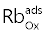, is converted to a reduced adsorbed form,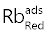: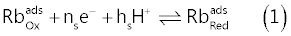Surface reaction (1) must be contrasted with the conventional volume reaction (2), which converts the oxidized Riboflavin into the solution,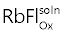, to the corresponding reduced form into the solution,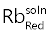: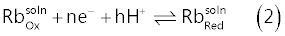There are a number of qualitative and quantitative aspects of Figure1A that can be used in order to detect if the CV currents are due to reaction (1) or (2). First, CV currents due to reaction (1) are predicted, after the CV peak has been overcome, to fall to the background (as in Figure 1A) when the adsorbed oxidized Riboflavin has been completely converted to the corresponding reduced form. This is not the case if the CV current is sustained by reaction (2), since the Riboflavin consumed at the electrode surface is replenished by a diffusional flux of the substance from the bulk of the solution (and, in abstract, the CV electrolysis current never reaches the background) [8,9]. On the other side, it can be demonstrated that the large currents in Figure 1A, could never be produced from reaction (2) at the low concentration of Riboflavin in the electrolyzed solution. Finally, the theory of CV predicts that the peak currents, ip, (both during the direct and inverse CV scans) due to the conventional volume reaction (2) increase with the square root of the scan rate v (i.e., ip ∝ v1/2) while peak currents due to reaction (1) increase proportionally to the scan rate (i.e., ip ∝ v) [8,9]. Plots of the peak’s currents, measured from voltammograms in Figure 1A, as a function of the scan rate are presented in Figure1B, from which there can be no doubt that CV currents in Figure 1A are essentially due to surface reaction (1). Stoichiometry of the surface electrode reaction It is useful, although not strictly required, that an electroanalytical method be based on an electrode reaction of known stoichiometry. First, in order to find the number, ns, of electrons consumed in reaction (1) the CV voltammograms in Figure1A will be confronted with calculated voltammograms simulated on the basis of an assumed model. Then, CV voltammograms acquired at a number of different pH will be employed in order to state the number of protons, hs, involved in the surface reaction (1). In order to calculate CV voltammograms to be confronted with the experimental ones, the model must specify, amongst other things, a mechanism for the process of adsorption of Riboflavin at the electrode surface because a number of different theoretical possibilities exist. A very useful and practical hypothesis is to imagine that Riboflavin is adsorbed onto the electrode surface exclusively in the course of the pre-electrolysis step during which the electrode surface is, to some degree, covered with a monolayer of the adsorbed substance. The degree of coverage of the electrode with Riboflavin is indicated with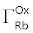(mol/cm2) and it is assumed that no further adsorption takes place in the very short time needed for the CV potential scan. During the CV scan,decreases and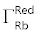increases as reaction (1) progresses, but the sumkeeps constant to a value, Γ*, which depends on the length of the pre-electrolysis time, on the efficiency of stirring and on potential of the working electrode during preelectrolysis. In other words, we have: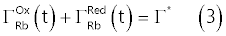In equation (3), we have explicitly stated that coveragesanddepend on time, ts, during the CV scan. This model of the adsorption process is called model of frozen adsorption , with reference to the fact that although the electrode surface may not be saturated during pre-electrolysis, no further adsorption is supposed to take place in the course of the electrochemical potential scan. In order to check if the frozen adsorption model was reasonable for describing the Riboflavin adsorption at the HMDE, an experiment was performed in which ten CV voltammograms, at 0.500 V/s, were acquired by performing in sequence ten CV cycles on a single mercury drop on which Riboflavin had been initially adsorbed from its solution during a short pre-electrolysis time (35 s at -â��0.15 V vs. Ag|AgCl|3·M·KCl). The ten CV voltammograms collected in the course of the ten CV cycles are shown in Figure 2. In abstract, if the frozen adsorption model is strictly verified, traces of the current measured in successive CV cycles should be perfectly superimposable, since the model predicts that, which is reduced during the direct scan, is simply regenerated during the inverse scan, and then all successive CV scans start with the mercury drop having the same Riboflavin coverage, i.e.,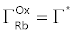. Figure 2 shows that this is not exactly the case for Riboflavin adsorption at the HMDE, but deviations are not dramatic in the light of the fact that a non negligible length of time has elapsed between the first and tenth CV cycle during which extra adsorption of Riboflavin could have taken place. On this basis the model of frozen adsorption was considered appropriate and was assumed for simulations. It has been mentioned above that, under the conditions adopted for acquiring the CV voltammograms in Figure 1A, the electrolysis current due to the volume reaction (2) is negligible because the concentration of Riboflavin is much below the threshold which can be detected by conventional Cyclic Voltammetry. This implies that the recorded faradic current, i (Ampere), which, by assumption, is exclusively due to the surface reaction (1), is connected to the electrode potential, E (volt), by the surface Butler- Volmer equation (4):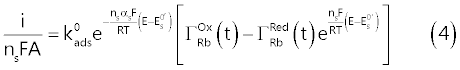Equation (4) is the fundamental relation which connects the electrolysis current to the instantaneous value of the electrode potential [9,12], embodying a number of parameters which characterize the surface reaction: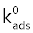= standard surface rate constant (s-1)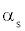= electron transfer coefficient;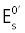= surface standard electrode potential of reaction (1) (volt); ns = number of electrons consumed by surface reaction (1); A = electrode area (cm2). Other symbols have their usual significance (i.e., T, F and R, represent respectively the temperature (K), the Faraday’s constant (C mol-1) and the gas constant (J mol-1 K-1)). Apart from that, the assumption of the model of the frozen adsorption imposes a constraint on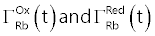which is expressed by relation (3). A very insidious item, for simulating the CV voltammograms, is the fact that the coverages, which appear in the surface Butler-Volmer equation (4), must be expressed as functions of the instantaneous concentration of Riboflavin at the electrode surface,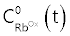and of its reduction product,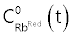In practice, this signifies that an adsorption isotherm, which connects the coverage of the electrode to the concentrations of Riboflavin and its reduction product into the solution, must be assumed. Assuming a Langmuir type isotherm [2,8,9], we obtain the following relations (5) and (6) which, in abstract, can be used to replace the coveragein the Butler-Volmer equation (4) with the concentrationsat the electrode surface: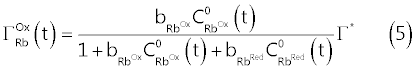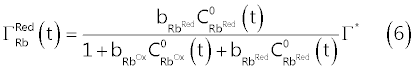In equations (5) and (6),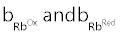represent the adsorption coefficients, respectively, of Riboflavin and its reduction product, and carry the dimensions of cm3/mol since concentrations, as conventional in electrochemical literature, are expressed in mol/cm3. On the basis of the above assumptions, an exact mathematical problem can be stated and solved to obtain predicted current → potential curves to be confronted with the experimental i → E traces [9,13]. The mathematical problem consists essentially into the solution of a system of partial differential equations (diffusion equations), to which the frozen adsorption model and Langmuir adsorption isotherm contribute the necessary boundary condition. We have obtained numerical solutions of the diffusion equations using the function < NDSolve > (Numerical Solution of Differential Equations) of Wolfram Mathematica . A distinctive feature of numerical solving of the diffusion equations is that values of all parameters which appear in equations (4), (5) and (6) must be specified in advance. Since our primary interest is in the coefficient ns, which specify the number of electrons in the surface reaction, and since, obviously, this coefficient is presumably a small integer, we adopted the following iterative strategy. For a given assumed value of ns, solutions of the diffusion equations, to be compared with experimental data, were obtained systematically varying the remaining parameters (i.e.,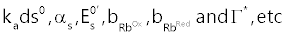.), around a central set of values which, on the basis of theoretical and empirical considerations based on a number of ad hoc experiments, were retained reasonable. In practice, for an assumed set of parameters the calculated i → E curves were visually compared with the experimental CV voltammograms and a new set of parameters, to be used in the successive iteration step, consciously identified on the basis of the detected differences between calculated and experimental CV voltammograms. This iterative procedure was continued until the fit between calculated and experimental voltammograms could no further be improved by modifying the parameters. At first sight this procedure may appear tedious due to the large number of parameters to be varied, but it becomes very fast once suitable code has been developed to take advantage of the graphical power of Wolfram Mathematica. On the other side, once the procedure is started it becomes very soon apparent that only when ns = 2 it is possible to obtain a reasonable fit of experimental CV voltammograms with the calculated ones. In Figure 3, experimental CV voltammograms of Figure 1A (solid curves) are confronted with the corresponding CV voltammograms calculated assuming ns = 2 and other parameters given in the caption to the Figure 3 (broken curves). It can be seen, from Figure 3, that, taking into account the number of assumptions made to develop a reasonably simple model of the adsorption process, the correspondence of calculated and experimental voltammograms is quite satisfying, so much so that we can now assume that adsorbed Riboflavin is reduced according to the surface reaction (11):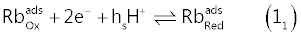The procedure employed to deduce the value of hs in reaction (11) is similar to the one employed for stating the value of ns, but the set of experimental CV voltammograms in Figure 4 (solid curves) acquired at a number of different pH are employed. In the present case, the parameter of interest, which incorporates the effect of pH on the CV voltammograms, is the standard formal electrode potential of the surface reaction (11),(volt). In fact, from the surface Nernst equation for reaction (1), the following relation (7) can be derived :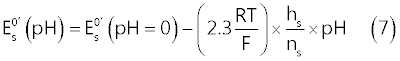In order to extract values ofat a number of different pH, experimental CV voltammograms in Figure 4 are simulated using the same procedure described above, systematically modifying, for each voltammogram, the value of, until the calculated voltammogram reproduced satisfyingly the experimental one.values deduced in the range 4.33 < pH < 9.2, from CV voltammograms in Figure 4 are presented in Table 1. In Figure 4, the broken curves represent the CV voltammograms simulated, at each pH, withvalues in Table 1 (and other parameters given in the legend of the figure). The very weak secondary peak which can be seen in Figure 4B, at about -0.6 V vs. Ag|AgCl, is due to reduction of adsorbed lumichrome (see Scheme 2) which, in a series of ad hoc experiments, was recognized as the main product of photodecomposition of Riboflavin under the experimental conditions of Figure 4. In Figure 5, it is presented a plot in which values of the ratio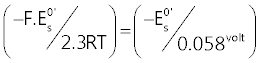, calculated fromin Table1 are plotted as a function of pH. It can be seen from Figure5 that experimental (-/ 0.058volt, pH) points approach, at low pH, a straight line of slope one, while, at high pH, they gradually move towards a line of slope 0.5. In the light of relation (7) this can be interpreted to signify that hs = 2 at low pH and becomes 1 in alkaline solutions. Then, the stoichiometry of the surface reaction (11) changes from: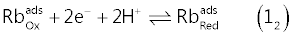to: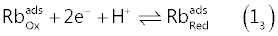concurrently with a change in pH of the electrolyzed solution from about four to nine. In other words, the reduced form of Riboflavin,, exists as two Acid-Base conjugate species, which can be indicated as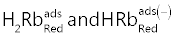, which are in equilibrium via the heterogeneous acid dissociation reaction (8):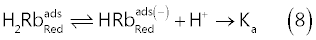The acid dissociation constant of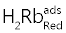can graphically be calculated as described in Figure5 (i.e., Ka = 10-6.5(5) ± 0.1). For convenience, results of the CV investigation of adsorption and reduction of Riboflavin at a mercury surface are synthesized in Figure 6. DP AdCSV external calibration plots of Riboflavin The opportunity of determining Riboflavin via AdCSV is a rather obvious consequence of the facts described in previous paragraphs. For this, however, Cyclic Voltammetry is not the most suitable electroanalytical technique, and it will be substituted with Differential Pulse Voltammetry (DPV). Figure 7A presents a sequence of DP voltammograms acquired at the HMDE from buffered standard solutions containing concentrations of Riboflavin between about 0.55 and 3.5 mg/l. Before each DP scan the mercury drop of the HMDE was enriched with adsorbed Riboflavin allowing a very short pre-electrolysis time (15 s at - 0.15 V vs. Ag|AgCl|3·M·KCl). The differential DP peak current, ip, measured in correspondence of maxima of DP voltammograms in Figure 7A, is plotted as a function of the Riboflavin concentration in order to obtain a calibration plot shown in Figure 7B. From Figure 7B, it is clear that the DP peak current depends linearly on the Riboflavin concentration and most of the experimental (ip, C) points are well fitted with a regression line which passes through the origin. However, a very noticeable fall of the response takes place when the concentration of Riboflavin in the electrolyzed solution is increased above about 3 mg/l. This is not unexpected since by increasing Riboflavin concentration the surface of the very small mercury drop becomes saturated and no further adsorption and increase of the response can take place by increasing the concentration of the analyte and/or the pre-electrolysis time. Since, for instrumental reasons, the pre-electrolysis time cannot be decreased under about 10 seconds, from Figure 7B we deduce that, under DP voltammetric conditions similar to those described in the legend of the figure, the concentration of the Riboflavin in the electrolyzed solution must not exceed about 2 mg/l in order to maintain a linear DP response. In Figure 8, a much lower range of Riboflavin concentrations (between about 17 and 116 μg/l) is explored. DP voltammograms in Figure 8A have been acquired under voltammetric conditions very similar to those used for acquiring DP voltammograms in Figure 7, except that a longer pre-electrolysis time (68 s) was used. By confronting DP voltammograms in Figure 7 and Figure 8, a very peculiar aspect of stripping techniques becomes apparent. Stripping techniques do not have, in abstract, a strictly definable limit of detection, since the concentration of analyte in the electrolyzed solution can be decreased, without decreasing response, by extending the pre-electrolysis time. For example, one can easily see that DP voltammograms in Figure 7A and Figure 8A expose very similar currents, even if solutions in Figure8 have Riboflavin concentrations which are order of magnitude lower than in Figure 7. Nevertheless, although a lower limit of detection of Riboflavin cannot strictly be stated, from the calibration plot in Figure8B a detection limit (expressed as the concentration of Riboflavin in the electrolysed solution) of 7 μg/l can be calculated, using the Hubaux and Vos approach  (under typical DP conditions using standard voltammetric equipment). Since the volume of electrolyzed solution during the acquisition of DP voltammograms in Figure 8A was 20.1 ml, the above figure implies that about 0.14 μg of Riboflavin can be assayed by DP AdCSV using a pre-electrolysis time of about 60-100 s (depending on the efficiency of stirring). These figures can be compared with corresponding values of the spectrophotometric method in  where linear calibration curves between 0.3 parts per million and 46.4 parts per million of Riboflavin are reported. DP AdCSV assay of Riboflavin in real samples by the standard additions calibration method Determination of an analyte in real samples by electroanalytical methods almost invariably takes place by employing the standard additions calibration technique , rather than being based on comparison of the measured response with a separately acquired external standard calibration function, as the ones presented in Figure 7B and Figure 8B. Because electrolysis currents generated by modern voltammetric techniques are so low and last for a very short time, reduction or oxidation of an analyte at the working electrode does not modify its bulk concentration. So much so, that the current → potential curve can be acquired countless times without the need of replacing the solution in the electrolysis cell with a new solution. Furthermore, the use of an HMDE as a working electrode allows, before each acquisition, the substitution of a new mercury drop for the old one, so that memory effects, resulting from previous acquisitions, are completely concealed and each acquisition, in abstract, reproduces exactly the previous one. These facts makes integration of the standard additions calibration procedure with electroanalytical techniques performed at the HMDE very convenient. In fact, several calibration points can be acquired across the standard additions curve simply by repeating the same acquisition on a new mercury drop after each standard addition of a standard solution of the analyte to the electrolyzed solution. Apart from this practical consideration, widespread use of the standard additions calibration technique also originates from the need of compensating for matrix effects on the measured electrolysis current which may arise from interactions of a variety of substances with the working electrode surface. However, standard additions can only correct for so called rotational matrix effects which consist in a change, from sample to sample, of the slope of the calibration curve . Translational matrix effects, consisting in a response incorporating a signal from extraneous interfering substances, which, usually, is mentioned as a background or baseline, are not corrected by the standard additions method and must be separately subtracted from the analytical response to avoid inaccuracies. From this point of view the DPV technique is ideally synergic with the standard additions calibration method, because the DP current is actually the difference between two currents measured, respectively, before and after the application of a short potential pulse of low amplitude, and most of the translational matrix effects are automatically compensated. This can be easily appreciated from DP voltammograms of Figure 7 and Figure 8 in which the DP current of Riboflavin ascend from a very low and regular background. Extrapolation of the baseline of a DP voltammogram in the range of potential occupied by the analyte response, as necessary for measuring the neat analytical signal, is, in general, an unambiguous process. The absence of translational effects on the Riboflavin DP response can also be judged from the fact that the regression lines in Figure 7B and Figure 8B pass through the origin. Nevertheless, the standard additions calibration principle prescribes that analytical results be derived by extrapolating the standard additions calibration curve, interpolating a number of experimental points, to zero response. Since extrapolation of a non linear standard additions plot is an unwise undertaking, a condition sine qua for the application of the method of standard additions is to prove that calibration plots are indeed linear. In this respect, the demonstration in Figure 7 and Figure 8, namely that calibration performed using standard solutions of Riboflavin gives straight regression lines passing through the origin, is the minimum evidence required for predicting an unbiased application of the standard additions method to Riboflavin determination in real samples. Obviously, one must be very vigilant, during the application of the internal standard additions method to the DP AdCSV assay of Riboflavin, that a suitable combination of pre-electrolysis time, initial concentration of Riboflavin in the electrolyzed solution and sizes of standard additions is selected in order to avoid the fall of response due to saturation of mercury drop with adsorbed Riboflavin. Guidelines on what may be a suitable combination of these factors can be deduced from Figure 7 and Figure 8. It is obviously impossible to demonstrate the applicability of the DP AdCSV technique via standard additions to the variety of samples on which an assay of Riboflavin may be required. However, since the procedure is essentially the same regardless of the nature of the sample, the symbolic determination of Riboflavin, in commercial tablets, containing a declared amount of Riboflavin, and a number of other compounds, presented in the next paragraph, will be sufficient to show the details of the suggested procedure. DP AdCSV assay of Riboflavin in tablets For this symbolic experiment, in which DP AdCSV determination of Riboflavin via standard additions calibration method is demonstrated, we use tablets each nominally containing, amongst other, 100 mg of Riboflavin. Tablets produced by a leader pharmaceutical company were selected in order to be reasonably confident that the average content of Riboflavin per tablet corresponded to the declared value. For the analysis, five tablets were finely grinded in a pestle and quantitatively transferred to 1 litre volumetric flask wrapped up in aluminum foil to protect Riboflavin from light. The volumetric flask was filled to the mark with distilled water previously alkalified to pH ≈ 10 with NaOH and the mixture was kept under stirring for about 1 hour in order to allow dissolution of Riboflavin from tablets. Then, 2.00 ml of this solution were extracted with a micropipette and transferred to a 250 ml volumetric flask (wrapped up in aluminum foil) which was filled to the mark with distilled water. Finally, 0.700 ml of this diluted solution were transferred to the electrolysis cell in which 20 ml of the electrolysis medium had been previously allotted (so that the initial volume of the electrolyzed solution is 20.70 ml). The electrolysis cell was carefully enveloped in aluminum foil to avoid Riboflavin decomposition during measurements. The electrolysis medium was simply made up by mixing in the electrolysis cell 18 ml distilled water with 2 ml of 1 M Acetic acid/ 1 M Sodium acetate (pH ≈ 4.6) buffer and dissolving into the mixture 2.5 g of solid NaClO4. The Riboflavin concentration transferred by the sample to the electrolysis medium will be called medium concentration of Riboflavin and is the most direct result to be obtained from the standard additions calibration method. For instance, the above operations have transferred to the electrolysis cell the content of 2.8â��10-5 tablets and, then, nominally 2.8â��10-5â��100 = 2.8Î�10-3 mg of Riboflavin. This corresponds to a nominal medium concentration of Riboflavin of 1000â��2.8/20.7 = 135 μg/l. Quantification, according to the method of standard additions, takes place by successively increasing the Riboflavin concentration in the electrolysis cell through measured additions of a standard Riboflavin solution. Each standard addition transfers to the electrolysis medium a concentration of Riboflavin which is called added concentration. In the present case we have used for Riboflavin quantification three 100 μl additions of a 40.70 mg/l Riboflavin standard solution. Each addition, then, transfers to the electrolyzed solution an added concentration of Riboflavin of 100Î�40.7/20.7 = 196.5 μg/l. The total concentration of Riboflavin in the electrolysis cell can be thought as the sum of the medium concentration and the added concentration. For each of the four calibration levels (corresponding to standard additions of 0 μl, 100 μl, 200 μl and 300 μl) two DP voltammograms were acquired in order to improve precision. Each DP voltammogram was acquired after a 28 s pre-electrolysis time using typical values for the other voltammetric parameters (see Figure 9A). In the standard additions plot of Figure9B, the measured peak currents, corrected for the increase in volume of the electrolyzed solution following the addition of standard solution, are reported as a function of the added concentration. It can be seen that the experimental (ip, AddedConc) points fall on a straight line. This is, in and by itself, a favourable event since it is the extrapolated intercept on the x-axis of the regression line through the experimental points that is of analytical significance in the method of standard additions. In the case of Figure 9B the regression line intercepts the added concentration axis at -137.5 μg/l and this is, except for sign, the determined medium concentration of Riboflavin in 20.70 ml of electrolyzed solution. The standard error of the regression line intercept on the x-axis, Sx, is evaluated from relation (9) to be 5 μg/l: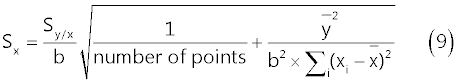In (9), Sy/x represents the standard deviation about regression, b is the slope of the regression line (nAÎ�l/μg) and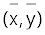is the centroid of all experimental (ip, AddedConc) points [16,18]. The Riboflavin content of the electrolysis cell is then 20.7Î�137.5/1000 = 2.85 ± 0.1 μg. Since this value is the content of 2.8Î�10-5 tablets, a single tablet will contain (2.85/1000)/2.8Î�10-5 = 101â��±â��5 mg Riboflavin/tablet, which compares favourably with the declared value (100 mg/tablet). Conclusion Riboflavin is strongly adsorbed on a mercury surface from solutions containing an excess of NaClO4 (as demonstrated by CV voltammograms acquired at various pH between about four and nine). The adsorbed Riboflavin can then be reduced by a negative-going potential scan via a surface reaction which consumes two electrons (see Figure 6). By consequence, Differential Pulse adsorptive cathodic stripping voltammetry at the HMDE, coupled to the standard additions calibration method, is a low-cost viable alternative to spectrometric and chromatographic methods for the determination of Riboflavin in a variety of samples. For this, under typical conditions, it is necessary to convey from the sample to the electrolysis cell (which contains 10 - 20 ml of a pH buffer plus ~ 1 M NaClO4) preferably at least ~ 0.5 μg of Riboflavin. Riboflavin is adsorbed (under controlled stirring) onto the mercury drop of the HMDE maintained at a potential of about -â��0.15 V vs. Ag|AgCl|3·M·KCl for an appropriate pre-electrolysis time (to be selected on the basis of Riboflavin concentration in the electrolysis medium) and, finally, forced stirring is stopped and a negative-going DP potential scan is applied. A dynamic linear range of Riboflavin concentration in the electrolysed solution extending from 17 μg/l to about 2 mg/l can be explored with typical DP settings and pre-electrolysis time between ~ 10 s and ~100 s (depending on available equipment and on stirring efficiency and working electrode potential during pre-electrolysis). The DP response of adsorbed Riboflavin is displaced toward more negative potentials by increasing the pH (see Table 1 and Figure 4) and this fact may be useful to avoid interferences by consciously selecting the pH of the electrolysis medium between about four and nine. References Dryhurst G, Kadish KM, Schelle F, Renneberg R (1982) Biological Electrochemistry.Volume 1, Academic Press Inc., London, UK. Wang J (2001) Analytical Electrochemistry. (2nd Edition), Wiley-VCH, New York, USA. Dogan-Topal B, Ozkan SA, Uslu B (2010) The Analytical Applications of Square Wave Voltammetry on Pharmaceutical Analysis. The Open Chemical and Biomedical Methods Journal 3: 56-73. Thomas FG, Henze G (2001) Introduction to voltammetric Analysis. CSIRO Publishing, Collingwood, Australia. Van Leeuwen HP, Buffle J, Lovric M (1992) Reactant Adsorption in Analytical Pulse Voltammetry: Methodology and Recommendations. Pure and Appl Chern 64: 1015-1028. Preedy VR (2013) B Vitamins and Folate: Chemistry, Analysis, Function and Effects. RSC Publishing, Cambridge, UK. Bartzatt R, Wol T (2014) Detection and Assay of Vitamin B-2 (Riboflavin) in Alkaline Borate Buffer with UV/Visible Spectrophotometry. International Scholarly Research Notices 2014: 1-7. Compton RG, Banks CE (2011) Understanding Voltammetry. (2nd Edition), Imperial College Press, London, UK. Bard AJ, Faulkner RL (2001) Electrochemical Methods, Fundamentals and Applications. Chapter 14 and references therein. John Wiley and Sons Inc., New York, USA. Malinausks A (2008) Electrochemical study of riboflavin adsorbed on a graphite electrode. Chemija 19: 1-3. Kakutani T, Katsho I, Senda M (1983) Electrochemical Oxidation and Reduction of Flavin-Adenine Dinucleotide Adsorbed on a Mercury Electrode Surface. Bull Chem Soc Jpn 56: 1761-1767. Sluyters-Rehbach M, Sluyters JH (1975) Some basic views on the influence of reactant adsorption on wave shapes in D.C., A.C. and Linear Sweeep Voltammetry. J Electroanal Chem 65: 831-841. Britz D (2005) Digital Simulation in Electrochemistry. Springer-Verlag, Berlin Heidelberg. http://www.wolfram.com/mathematica/ http://reference.wolfram.com/mathematica/ Hubaux A, Vos G (1970) Decision and detection limits for linear calibration curves. Anal Chem 42: 849-855. Harvey D (2000) Modern Analytical Chemistry. McGaw-Hill Higher Education, Boston, USA. Ellison LRS, Thompson M (2008) Standard Addition: Myth and reality. Analyst 133: 992-997. Miller JN, Miller JC (2010) Statistics and Chemometrics for Analytical Chemistry. (6th Edition), Prentice Hall, Harlow, Essex, UK.

#### Tables and Figures at a glanceTable 1

#### Figures at a glance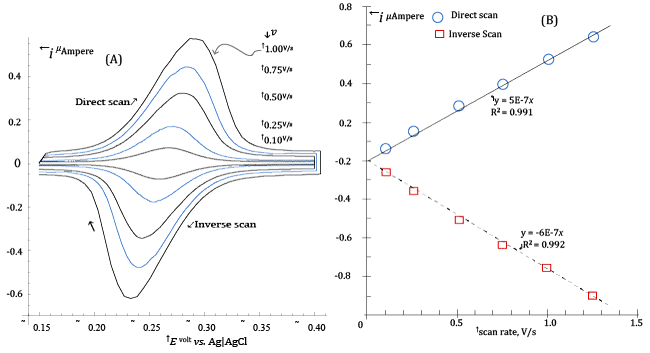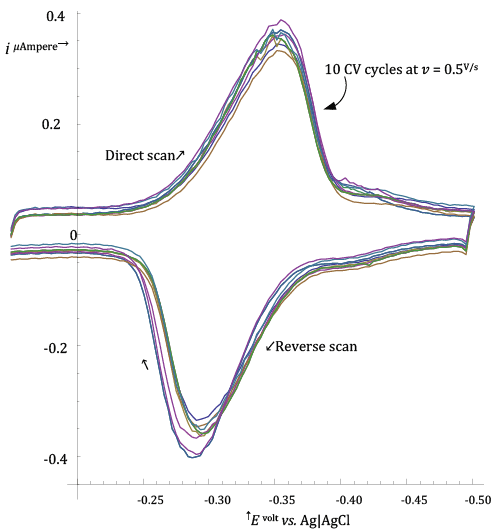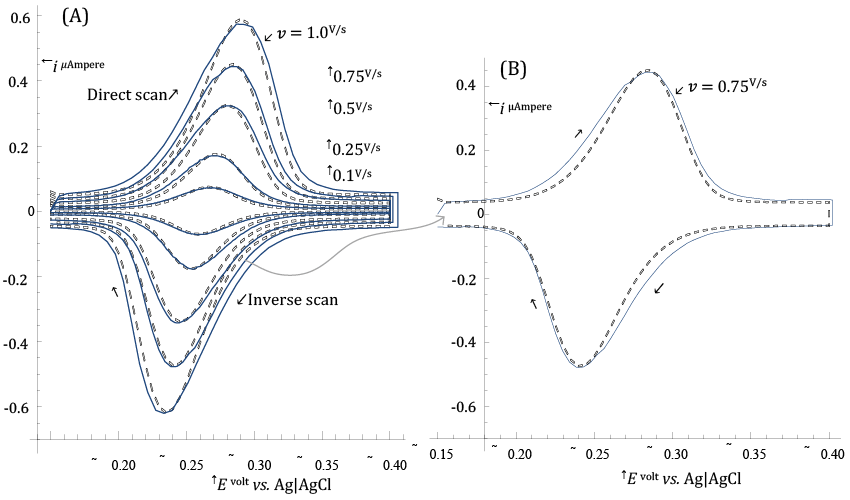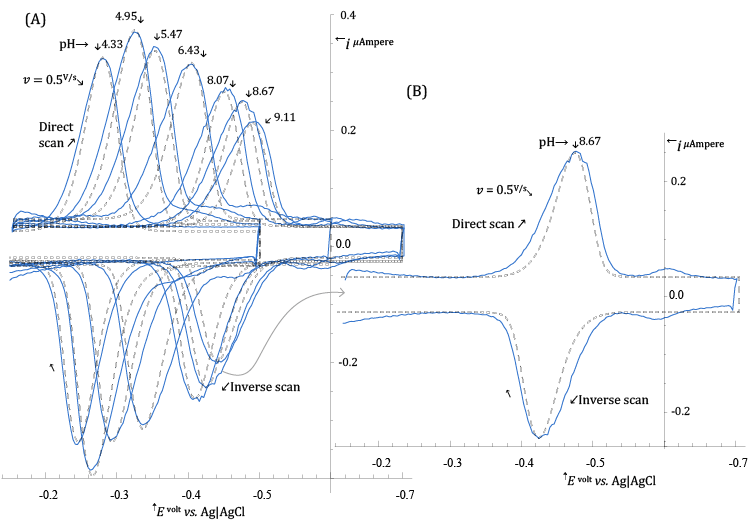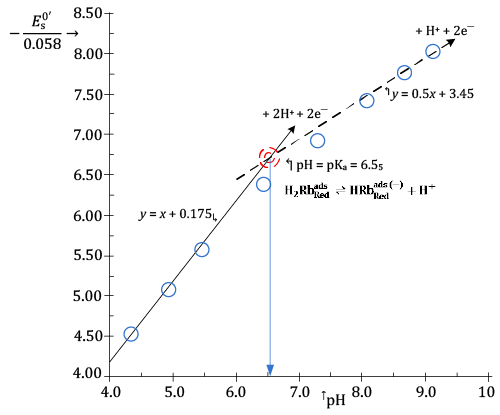Figure 1 Figure 2 Figure 3 Figure 4 Figure 5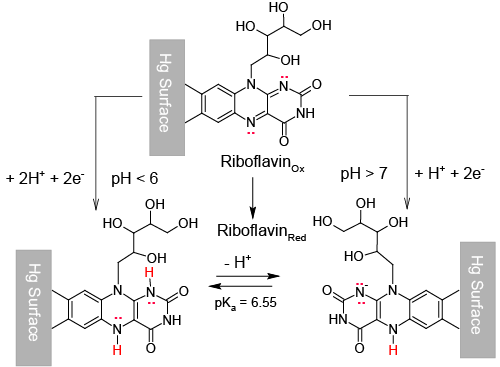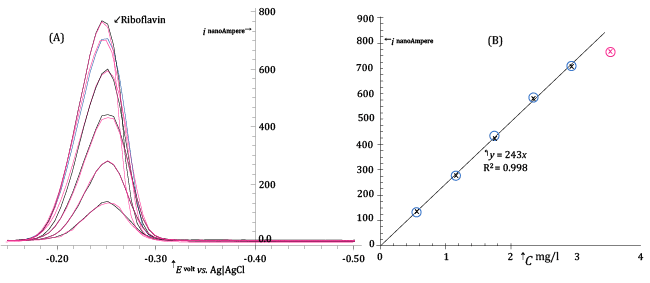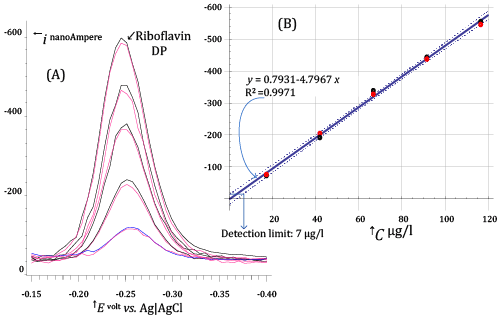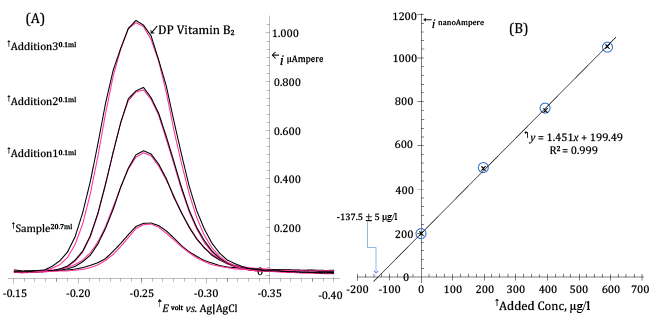Figure 6 Figure 7 Figure 8 Figure 9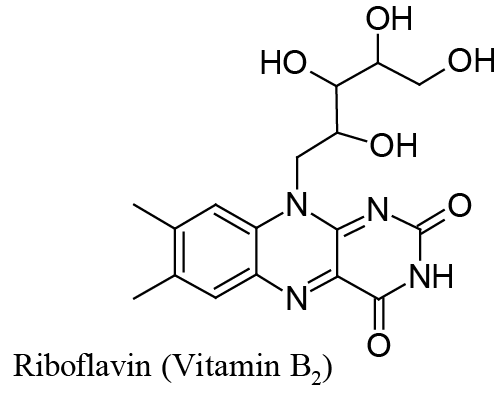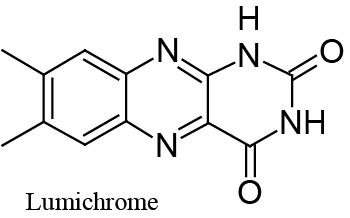Scheme 1 Scheme 2
Select your language of interest to view the total content in your interested language

### Article Usage

• Total views: 13321
• [From(publication date):
December-2015 - Aug 04, 2021]
• Breakdown by view type
• HTML page views : 9370Can't read the image? click here to refresh
###### Peer Reviewed Journals

Make the best use of Scientific Research and information from our 700 + peer reviewed, Open Access Journals